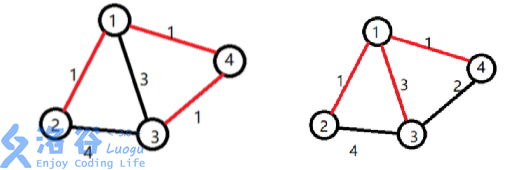P3959 宝藏

• 5.2K通过
• 25.6K提交
• 题目提供者 CCF_NOI
• 评测方式 云端评测
• 标签 枚举,暴力 状态压缩,状压 贪心 NOIp提高组 2017
• 难度 省选/NOI-
• 时空限制 1000ms / 256MB
• 提示：收藏到任务计划后，可在首页查看。

题目描述

参与考古挖掘的小明得到了一份藏宝图，藏宝图上标出了 $n$ 个深埋在地下的宝藏屋， 也给出了这 $n$ 个宝藏屋之间可供开发的$m$ 条道路和它们的长度。

小明决心亲自前往挖掘所有宝藏屋中的宝藏。但是，每个宝藏屋距离地面都很远， 也就是说，从地面打通一条到某个宝藏屋的道路是很困难的，而开发宝藏屋之间的道路 则相对容易很多。

小明的决心感动了考古挖掘的赞助商，赞助商决定免费赞助他打通一条从地面到某 个宝藏屋的通道，通往哪个宝藏屋则由小明来决定。

在此基础上，小明还需要考虑如何开凿宝藏屋之间的道路。已经开凿出的道路可以 任意通行不消耗代价。每开凿出一条新道路，小明就会与考古队一起挖掘出由该条道路 所能到达的宝藏屋的宝藏。另外，小明不想开发无用道路，即两个已经被挖掘过的宝藏 屋之间的道路无需再开发。

新开发一条道路的代价是：

$$\mathrm{L} \times \mathrm{K}$$

L代表这条道路的长度，K代表从赞助商帮你打通的宝藏屋到这条道路起点的宝藏屋所经过的 宝藏屋的数量（包括赞助商帮你打通的宝藏屋和这条道路起点的宝藏屋） 。

请你编写程序为小明选定由赞助商打通的宝藏屋和之后开凿的道路，使得工程总代 价最小，并输出这个最小值。

输入输出格式

输入格式：

第一行两个用空格分离的正整数 $n,m$，代表宝藏屋的个数和道路数。

接下来 $m$ 行，每行三个用空格分离的正整数，分别是由一条道路连接的两个宝藏 屋的编号（编号为 $1-n$），和这条道路的长度 $v$。

输出格式：

一个正整数，表示最小的总代价。

输入输出样例

输入样例#1： 复制
4 5
1 2 1
1 3 3
1 4 1
2 3 4
3 4 1

输出样例#1： 复制
4
输入样例#2： 复制
4 5
1 2 1
1 3 3
1 4 1
2 3 4
3 4 2  
输出样例#2： 复制
5

说明【样例解释1】

小明选定让赞助商打通了$1$ 号宝藏屋。小明开发了道路 $1 \to 2$，挖掘了 $2$ 号宝 藏。开发了道路 $1 \to 4$，挖掘了 $4$ 号宝藏。还开发了道路 $4 \to 3$，挖掘了$3$号宝 藏。工程总代价为：$1 \times 1 + 1 \times 1 + 1 \times 2 = 4$

【样例解释2】

小明选定让赞助商打通了$1$ 号宝藏屋。小明开发了道路 $1 \to 2$，挖掘了 $2$ 号宝 藏。开发了道路 $1 \to 3$，挖掘了 $3$ 号宝藏。还开发了道路 $1 \to 4$，挖掘了$4$号宝 藏。工程总代价为：$1 \times 1 + 3 \times 1 + 1 \times 1 = 5$

【数据规模与约定】

对于$20\%$的数据： 保证输入是一棵树，$1 \le n \le 8$，$v \le 5000$ 且所有的 $v$都相等。

对于 $40\%$的数据： $1 \le n \le 8$，$0 \le m \le 1000$，$v \le 5000$ 且所有的$v$都相等。

对于$70\%$的数据： $1 \le n \le 8$，$0 \le m \le 1000$，$v \le 5000$

对于$100\%$的数据： $1 \le n \le 12$，$0 \le m \le 1000$，$v \le 500000$

提示
标程仅供做题后或实在无思路时参考。
请自觉、自律地使用该功能并请对自己的学习负责。
如果发现恶意抄袭标程，将按照I类违反进行处理。